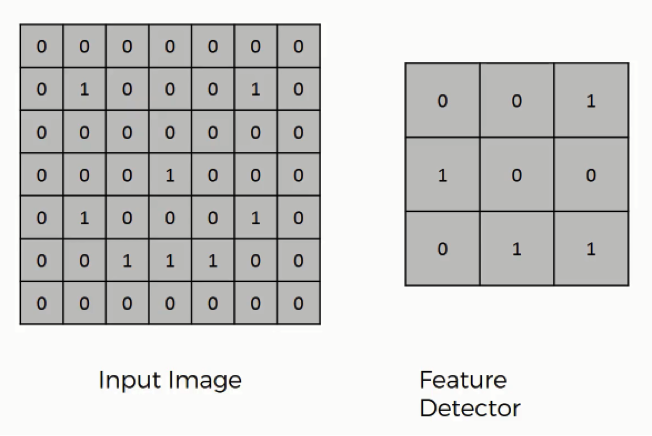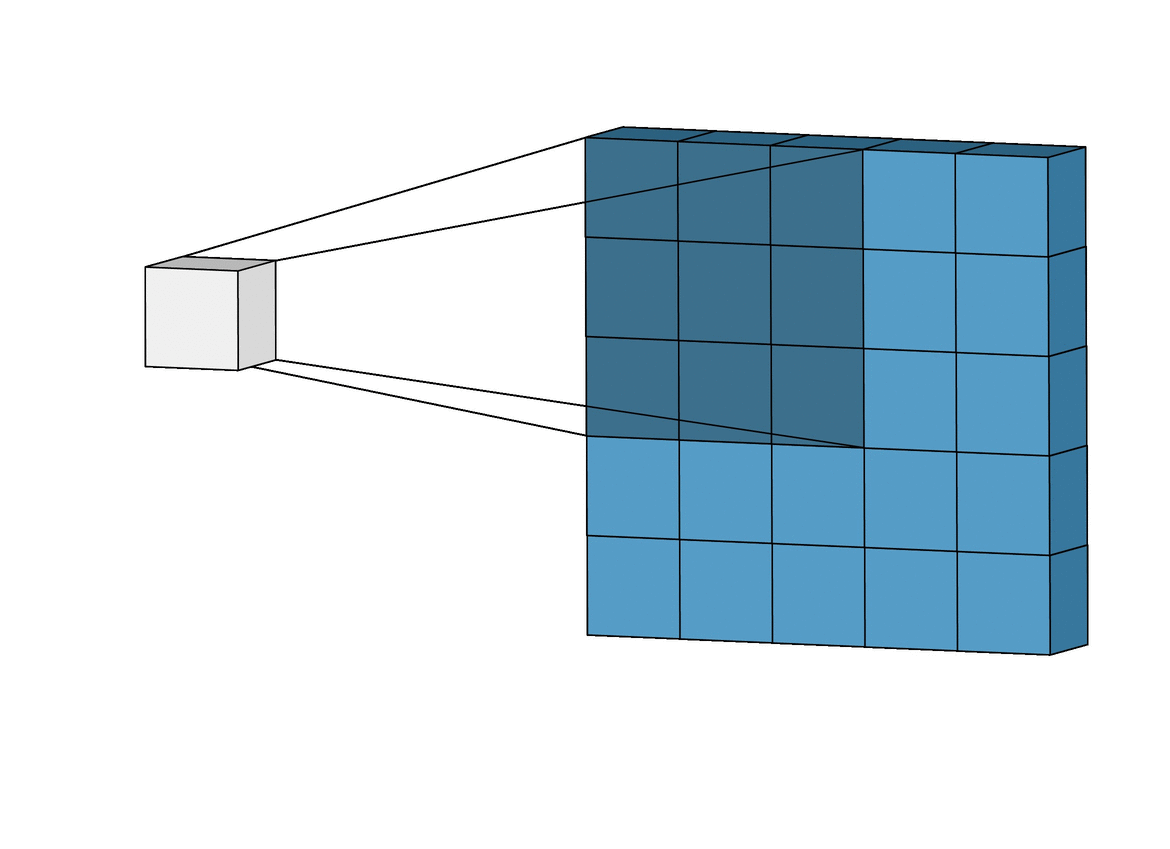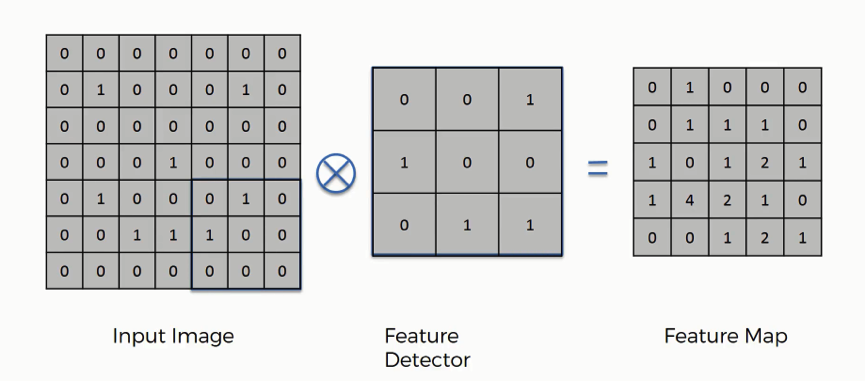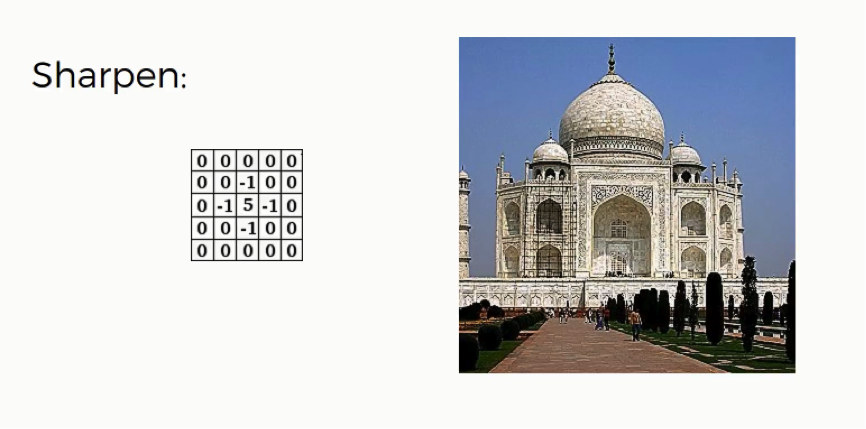# What Are Convolutions in Deep Learning?

Hey - Nick here! This page is a free excerpt from my \$199 course Python for Finance, which is 50% off for the next 50 students.

In the last section of this course, you had your first introduction to convolutional neural networks.

You learned the types of problems they're used to solve, their history (and the influence of Yann Lecun), and an example of an image that would be difficult to classify using an artificial neural network.

You have yet to see why convolutional neural networks have earned their name. Their name comes from the first step in building a convolutional neural network - convolution.

This tutorial will explain to you what convolution means and why it is important when building and training convolutional neural networks.

## What is a Convolution?

A convolution is a mathematical function that is defined as follows:Note that this is an integration with respect to `tau`, not with respect to `t`.

While it might not be clear from the equation itself, the convolution function is used to express how the shape of one function is modified by the other. The convolution function has applications in probability, statistics, differential equations, and - of course - convolutional neural networks in deep learning.

## Convolutions As The First Step in Convolutional Neural Networks

Convolutions are used as the first step in building a convolutional neural network. More specifically, they are used to transform an `input image` into a `feature map` using a `feature detector`.

Each of these items - the `input image`, the `feature detector`, and the `feature map` are arrays.

In terms of vocabulary, it should be noted that there are other terms for the items described above. The `feature detector` is also sometimes called the `kernel` or the `filter`. Similarly, the `feature map` is sometimes called the `convolved feature` or `activation map`.

Let's start by considering the two inputs in a convolution - the `input image` and the `feature detector`. They look like this:The `feature detector` is often a `3x3` matrix, although it can also be a `5x5` matrix or a `7x7` matrix (or even other variants). We will stick with the `3x3` example in this tutorial.

The way that the `input image` and the `feature detector` are integrated to generate the `feature map` are as follows:

• The `feature detector` is overlaid in the top-left corner of the `input image`.
• The number of cells that match between the `input image` and the `feature detector` are counted.
• This number is added to the top-left cell of the `feature map`.
• The `feature detector` is moved to the right by one column, and the process is repeated. Once the `feature detector` has been moved all the way to the right of the image, it is shifted down by one row.

Here is a visual example that is very helpful in understanding how convolution is used to generate the `feature map`:And here is the finalized feature map from our previous example:Using the example above, work through a few example placements to make sure that you understand how the convolution calculations are performed!

## Strides and Convolutions

In the example that we worked through earlier in this tutorial, we moved the `feature detector` by one column every iteration. We also moved it down one row once it reached the end of its row.

This value of `1` is called the `stride` of the convolution, and it doesn't need to be `1`. You could use a `stride` value of `1`, `2`, `3`, or higher - especially when working with larger images. It is generally considered convention to use a stride value of `2` when building and training convolutional neural networks.

We will revisit `stride` values later in this tutorial.

## Interpreting A Feature Map

You saw earlier in this tutorial that convolution can be used to generate a `feature map`, like this:How should you interpret the output of this convolution?

Well, this convolution process serves two main purposes:

• It reduces the size of the image: as you can see, the `feature map` is smaller than the `input image`. This makes it easier to process. Our iterations will run faster as a result.
• It allows the neural network to focus on specific features in an image: for facial recognition, these features might include a nose or an eye

In practice, many different feature maps are generated within a convolutional neural network to account for the various features you're looking for in an image. The following image illustrates this nicely:This will become more clear as we build and train a real convolutional neural network later in this course.

## Final Thoughts

This tutorial introduced you to the convolution function and its role in building convolutional neural networks.

Here is a brief summary of what we discussed in this tutorial:

• The mathematical definition of a convolution
• How convolutions are combined with matrix overlays to act as the first step in building a convolutional neural network
• The role of an `input image`, a `feature detector`, and a `feature map` in a convolutional neural network
• What a `stride` means when training a convolutional neural network
• How to interpret a convolutional neural network's `feature map`